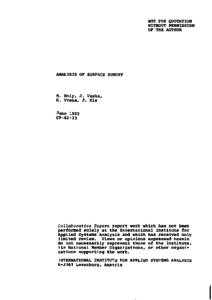# Analysis of Surface Runoff

Holy, M., Vaska, J., Vrana, K., & Mls, J. (1982). Analysis of Surface Runoff. IIASA Collaborative Paper. IIASA, Laxenburg, Austria: CP-82-033Preview Text CP-82-033.pdf Download (882kB) | Preview

## Abstract

A mathematical model of surface runoff is presented which is of use in building a model of erosion processes. The method used for deriving the conceptual model of surface runoff is based on the mathematical expression of the basic laws of movement of water -- the equation of continuity and the equation of motion. Both equations form a system of nonlinear partial differential equations with two unknown functions expressing the depth and velocity of the movement of water along the slope, in dependence on their location on the slope, and time. The input variables of the model are the intensity and direction of the impinging raindrops, the intensity of infiltration and the physical characteristics of the slope (gradient, length and properties of soil surface). Extensive laboratory experiments have been carried out to determine the functional dependence of tangential stress on the depth and rate of runoff from different types of soil surfaces.

Further, the conceptual model of surface runoff has been simplified to a kinematic one by using a simple relation between depth and rate of surface runoff instead of the equation of motion. Two empirical parameters of this relation have been determined by using data from the above mentioned laboratory experiments during calibration of the kinematic model. The kinematic model is recommended because of its simplicity with regard to simulation of the surface runoff formation from individual slopes within the watershed.

The model is a multipurpose one. It may be used either for hydrological purposes (simulation of surface runoff characteristics) or for soil conservation purposes. The model outputs are surface characteristics (depth, velocity, rate). It is possible by comparing the surface runoff velocity with the critical nonscouring velocity for given field conditions to determine the critical slope length which is the basis for planning efficient soil conservation measures.

Item Type: Monograph (IIASA Collaborative Paper) Resources and Environment Area (REN) IIASA Import 15 Jan 2016 01:52 27 Aug 2021 17:11 https://pure.iiasa.ac.at/2085View Item